# NCERT Solutions for Class 11 Chemistry Classification of Elements and Periodicity in Properties Part-2## myCBSEguide App

Complete Guide for CBSE Students

NCERT Solutions, NCERT Exemplars, Revison Notes, Free Videos, CBSE Papers, MCQ Tests & more.

NCERT solutions for Class 11 Chemistry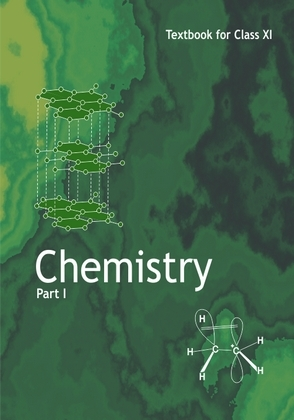## NCERT Class 11 Chemistry Chapter wise Solutions

• Chapter 1 – Some Basic Concepts of Chemistry
• Chapter 2 – Structure of Atom
• Chapter 3 – Classification of Elements and Periodicity in Properties
• Chapter 4 – Chemical Bonding and Molecular Structure
• Chapter 5 – States of Matter
• Chapter 6 – Thermodynamics
• Chapter 7 – Equilibrium
• Chapter 8 – Redox Reactions
• Chapter 9 – Hydrogen
• Chapter 10 – The s-Block Elements
• Chapter 11 – The p-Block Elements
• Chapter 12 – Organic Chemistry – Some Basic Principles and Techniques
• Chapter 13 – Hydrocarbons
• Chapter 14 – Environmental Chemistry

## NCERT Solutions for Class 11 Chemistry Classification of Elements and Periodicity in Properties Part-2

Class 11 Chemistry Classification of Elements and Periodicity in Properties Part-2

21. Would you expect the second electron gain enthalpy of O as positive, more negative or less negative than the first? Justify your answer.

22. What is the basic difference between the terms electron gain enthalpy and electro-negativity?

23. How would you react to the statement that the electro-negativity of N on Pauling scale is 3.0 in all the nitrogen compounds?

24. Describe the theory associated with the radius of an atom as it

(a) gains an electron

(b) loses an electron

25. Would you expect the first ionization enthalpies for two isotopes of the same element to be the same or different? Justify your answer.

26. What are the major differences between metals and non-metals?

27. Use the periodic table to answer the following questions.

(a) Identify an element with five electrons in the outer subshell.

(b) Identify an element that would tend to lose two electrons.

(c) Identify an element that would tend to gain two electrons.

(d) Identify the group having metal, non-metal, liquid as well as gas at the room temperature.

28. The increasing order of reactivity among group 1 elements is Li < Na < K < Rb <Cs whereas that among group 17 elements is F > CI > Br > I. Explain.

29. Write the general outer electronic configuration of s-, p-, d- and f- block elements.

30. Assign the position of the element having outer electronic configuration

(i)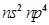for n = 3

(ii)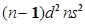for n = 4, and

(iii)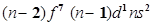for n = 6, in the periodic table.

31. The first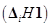and the second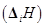ionization enthalpies (in kJ mol-1) and the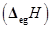electron gain enthalpy (in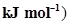of a few elements are given below: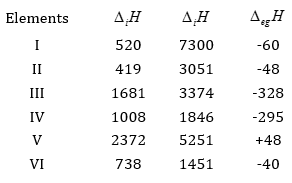Which of the above elements is likely to be:

(a) the least reactive element.

(b) the most reactive metal.

(c) the most reactive non-metal.

(d) the least reactive non-metal.

(e) the metal which can form a stable binary handle of the formula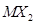, (X=halogen).

(f) the metal which can form a predominantly stable covalent halide of the formula MX (X=halogen)?

32. Predict the formula of the stable binary compounds that would be formed by the combination of the following pairs of elements.

(a) Lithium and oxygen

(b) Magnesium and nitrogen

(c) Aluminium and iodine

(d) Silicon and oxygen

(e) Phosphorus and fluorine

(f) Element 71 and fluorine

33. In the modern periodic table, the period indicates the value of:

(a) Atomic number

(b) Atomic mass

(c) Principal quantum number

(d) Azimuthal quantum number.

34. Which of the following statements related to the modern periodic table is incorrect?

(a) The p-block has 6 columns, because a maximum of 6 electrons can occupy all the orbitals in a p-shell.

(b) The d-block has 8 columns, because a maximum of 8 electrons can occupy all the orbitals in a d-subshell.

(c) Each block contains a number of columns equal to the number of electrons that can occupy that subshell.

(d) The block indicates value of azimuthal quantum number (l ) for the last subshell that received electrons in building up the electronic configuration.
35. Anything that influences the valence electrons will affect the chemistry of the element. Which one of the following factors does not affect the valence shell?

(a) Valence principal quantum number (n)

(b) Nuclear charge (Z)

(c) Nuclear mass

(d) Number of core electrons.

36. The size of isoelectronic species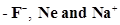is affected by

(a) Nuclear charge (Z )

(b) Valence principal quantum number (n)

(c) Electron-electron interaction in the outer orbitals

(d) None of the factors because their size is the same.

37. Which one of the following statements is incorrect in relation to ionization enthalpy?

(a) Ionization enthalpy increases for each successive electron.

(b) The greatest increase in ionization enthalpy is experienced on removal of electron from core noble gas configuration.

(c) End of valence electrons is marked by a big jump in ionization enthalpy.

(d) Removal of electron from orbitals bearing lower n value is easier than from orbital having higher n value.

38. Considering the elements B, Al, Mg, and K, the correct order of their metallic character is:

(a) B > Al > Mg > K

(b) Al > Mg > B > K

(c) Mg > Al > K > B

(d) K > Mg > Al > B

39. Considering the elements B, C, N, F, and Si, the correct order of their non-metallic character is:

(a) B > C > Si > N > F

(b) Si > C > B > N > F

(c) F > N > C > B > Si

(d) F > N > C > Si > B

40. Considering the elements F, Cl, O and N, the correct order of their chemical reactivity in terms of oxidizing property is:

(a) F > Cl > O > N

(b) F > O > Cl > N

(c) Cl > F > O > N

(d) O > F > N > Cl

## NCERT Solutions for Class 11 Chemistry Chapter 3  Classification of Elements and Periodicity in Properties Part-2

NCERT Solutions Class 11 Chemistry Classification of Elements and Periodicity in Properties Part-2 PDF (Download) Free from myCBSEguide app and myCBSEguide website. Ncert solution class 11 Chemistry includes text book solutions from both part 1 and part 2. NCERT Solutions for CBSE Class 11 Chemistry have total 14 chapters. 11 Chemistry NCERT Solutions in PDF for free Download on our website. Ncert Chemistry class 11 solutions PDF and Chemistry ncert class 11 PDF solutions with latest modifications and as per the latest CBSE syllabus are only available in myCBSEguide

## NCERT Solutions for Science Class 3rd to 12th

To download NCERT Solutions for class 11 Chemistry, Physics, Biology, History, Political Science, Economics, Geography, Computer Science, Home Science, Accountancy, Business Studies and Home Science; do check myCBSEguide app or website. myCBSEguide provides sample papers with solution, test papers for chapter-wise practice, NCERT solutions, NCERT Exemplar solutions, quick revision notes for ready reference, CBSE guess papers and CBSE important question papers. Sample Paper all are made available through the best app for CBSE students and myCBSEguide.com website.## Test Generator

Create Papers with your Name & Logo